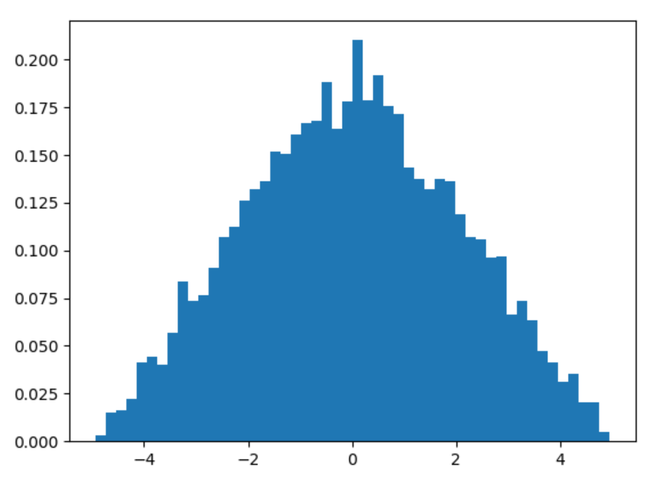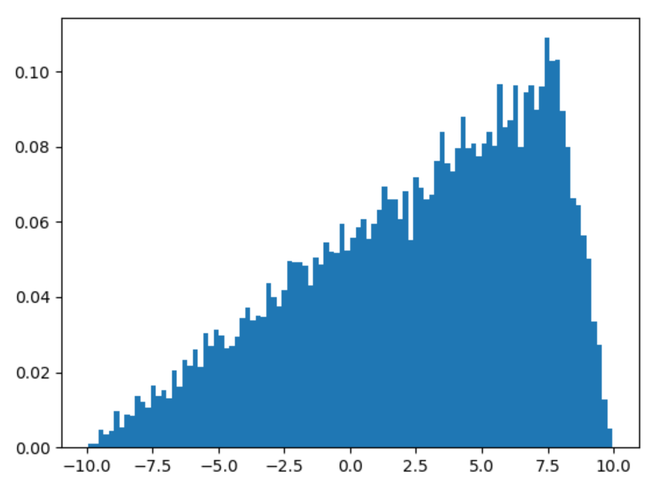# numpy.random.triangular() in Python

• Last Updated : 18 Aug, 2020

With the help of numpy.random.triangular() method, we can get the random samples from triangular distribution from interval [left, right] and return the random samples by using this method.

Syntax : numpy.random.triangular(left, mode, right, size=None)

Attention geek! Strengthen your foundations with the Python Programming Foundation Course and learn the basics.

To begin with, your interview preparations Enhance your Data Structures concepts with the Python DS Course. And to begin with your Machine Learning Journey, join the Machine Learning - Basic Level Course

Parameters :

1) left – lower limit of the triangle.

2) mode – peak value of the distribution.

3) right – upper limit of the triangle.

4) size – total number of samples required.

Return : Return the random samples as numpy array.

Example #1 :

In this example we can see that by using numpy.random.triangular() method, we are able to get the random samples of triangular distribution and return the numpy array.

## Python3

 `# import numpy``import` `numpy as np``import` `matplotlib.pyplot as plt`` ` `# Using triangular() method``gfg ``=` `np.random.triangular(``-``5``, ``0``, ``5``, ``5000``)`` ` `plt.hist(gfg, bins ``=` `50``, density ``=` `True``)``plt.show()`

Output :Example #2 :

## Python3

 `# import numpy``import` `numpy as np``import` `matplotlib.pyplot as plt`` ` `# Using triangular() method``gfg ``=` `np.random.triangular(``-``10``, ``8``, ``10``, ``15000``)`` ` `plt.hist(gfg, bins ``=` `100``, density ``=` `True``)``plt.show()`

Output :My Personal Notes arrow_drop_up